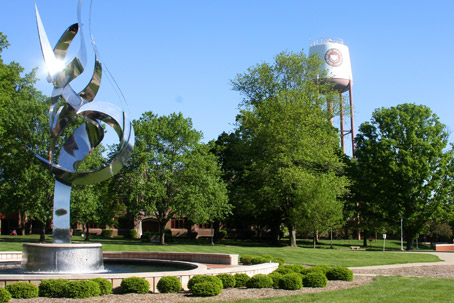# David L. FinnDr. David Finn specializes in partial differential equations, differential geometry, and the calculus of variations. His research applies these areas of expertise to mathematical physics, geometric modeling, and imaging science. He led a multi-year undergraduate research project that used these concepts to model the shape of a cookie at different stages of the baking process. Dr. Finn also co-leads the Accelerated Math Physics program, an intensive summer experience that allows outstanding incoming freshmen to complete several credits of calculus and physics in just five weeks. A native of the Boston area, he is a diehard Red Sox and Celtics fan. Check out his personal web page.

## Teaching Interests

• Calculus
• Differential equations
• Vector calculus
• Tensor calculus
• Riemannian geometry
• Partial differential equations

## Research Interests

• Applications of nonlinear differential equations in mathematical physics and geometry
• Modeling the baking process through continuum mechanics and material science
• Partial differential equations
• Differential geometry
• Calculus of variations
• Applications to physics and geometry

## Select Publications & Presentations

• “Modeling the Shape of a Cookie and Modeling the Baking Process,” Presented at a variety of conferences and symposiums, 2006-2012